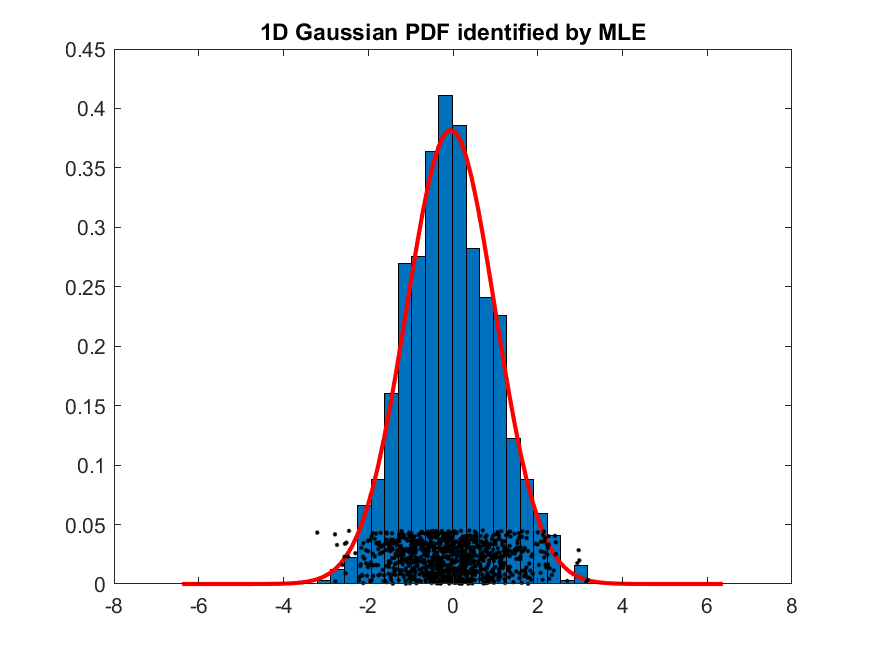4-3 PDF Modeling for 1D Gaussian

Mathematical treatment can be found here.

Here is an example of MLE for 1D Gaussian PDF modeling:

Example 1: mle4gaussian01.mdataNum=1000; x = randn(dataNum, 1); plotOpt=1; gPrm=gaussianMle(x, plotOpt); title('1D Gaussian PDF identified by MLE');Data Clustering and Pattern Recognition (資料分群與樣式辨認)